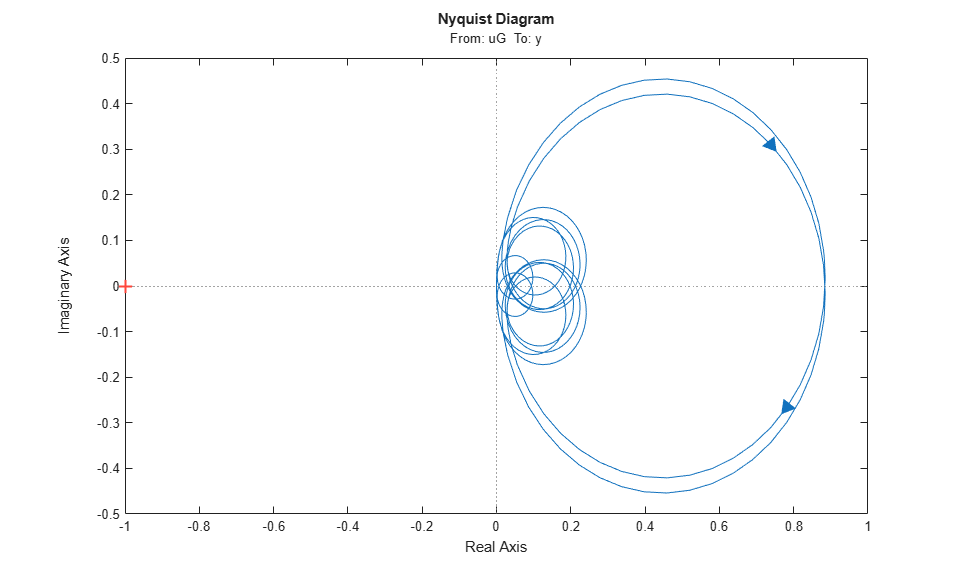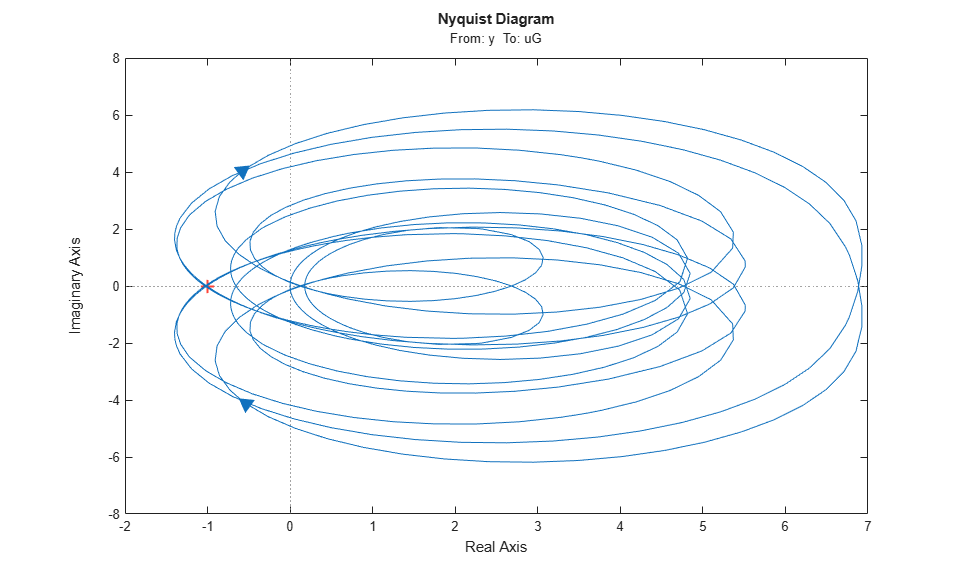# Vibration Control in Flexible Beam

This example shows how to tune a controller for reducing vibrations in a flexible beam.

### Model of Flexible Beam

Figure 1 depicts an active vibration control system for a flexible beam.Figure 1: Active control of flexible beam

In this setup, the actuator delivering the forceand the velocity sensor are collocated. We can model the transfer function from control inputto the velocityusing finite-element analysis. Keeping only the first six modes, we obtain a plant model of the formwith the following parameter values.

% Parameters xi = 0.05; alpha = [0.09877, -0.309, -0.891, 0.5878, 0.7071, -0.8091]; w = [1, 4, 9, 16, 25, 36]; 

The resulting beam model foris given by

% Beam model G = tf(alpha(1)^2*[1,0],[1, 2*xi*w(1), w(1)^2]) + ... tf(alpha(2)^2*[1,0],[1, 2*xi*w(2), w(2)^2]) + ... tf(alpha(3)^2*[1,0],[1, 2*xi*w(3), w(3)^2]) + ... tf(alpha(4)^2*[1,0],[1, 2*xi*w(4), w(4)^2]) + ... tf(alpha(5)^2*[1,0],[1, 2*xi*w(5), w(5)^2]) + ... tf(alpha(6)^2*[1,0],[1, 2*xi*w(6), w(6)^2]); G.InputName = 'uG'; G.OutputName = 'y'; 

With this sensor/actuator configuration, the beam is a passive system:

isPassive(G) 
ans = logical 1 

This is confirmed by observing that the Nyquist plot ofis positive real.

nyquist(G)### LQG Controller

LQG control is a natural formulation for active vibration control. The LQG control setup is depicted in Figure 2. The signalsandare the process and measurement noise, respectively.Figure 2: LQG control structure

First use lqg to compute the optimal LQG controller for the objectivewith noise variances:[a,b,c,d] = ssdata(G); M = [c d;zeros(1,12) 1]; % [y;u] = M * [x;u] QWV = blkdiag(b*b',1e-2); QXU = M'*diag([1 1e-3])*M; CLQG = lqg(ss(G),QXU,QWV); 

The LQG-optimal controller CLQG is complex with 12 states and several notching zeros.

size(CLQG) 
State-space model with 1 outputs, 1 inputs, and 12 states. 
bode(G,CLQG,{1e-2,1e3}), grid, legend('G','CLQG')Use the general-purpose tuner systune to try and simplify this controller. With systune, you are not limited to a full-order controller and can tune controllers of any order. Here for example, let's tune a 2nd-order state-space controller.

C = ltiblock.ss('C',2,1,1); 

Build a closed-loop model of the block diagram in Figure 2.

C.InputName = 'yn'; C.OutputName = 'u'; S1 = sumblk('yn = y + n'); S2 = sumblk('uG = u + d'); CL0 = connect(G,C,S1,S2,{'d','n'},{'y','u'},{'yn','u'}); 

Use the LQG criterionabove as sole tuning goal. The LQG tuning goal lets you directly specify the performance weights and noise covariances.

R1 = TuningGoal.LQG({'d','n'},{'y','u'},diag([1,1e-2]),diag([1 1e-3])); 

Now tune the controller C to minimize the LQG objective.

[CL1,J1] = systune(CL0,R1); 
Final: Soft = 0.478, Hard = -Inf, Iterations = 39 

The optimizer found a 2nd-order controller with. Compare with the optimalvalue for CLQG:

[~,Jopt] = evalGoal(R1,replaceBlock(CL0,'C',CLQG)) 
Jopt = 0.4673 

The performance degradation is less than 5%, and we reduced the controller complexity from 12 to 2 states. Further compare the impulse responses fromtofor the two controllers. The two responses are almost identical. You can therefore obtain near-optimal vibration attenuation with a simple second-order controller.

T0 = feedback(G,CLQG,+1); T1 = getIOTransfer(CL1,'d','y'); impulse(T0,T1,5) title('Response to impulse disturbance d') legend('LQG optimal','2nd-order LQG')### Passive LQG Controller

We used an approximate model of the beam to design these two controllers. A priori, there is no guarantee that these controllers will perform well on the real beam. However, we know that the beam is a passive physical system and that the negative feedback interconnection of passive systems is always stable. So ifis passive, we can be confident that the closed-loop system will be stable.

The optimal LQG controller is not passive. In fact, its relative passive index is infinite becauseis not even minimum phase.

getPassiveIndex(-CLQG) 
ans = Inf 

This is confirmed by its Nyquist plot.

nyquist(-CLQG)Using systune, you can re-tune the second-order controller with the additional requirement thatshould be passive. To do this, create a passivity tuning goal for the open-loop transfer function from yn to u (which is). Use the "WeightedPassivity" goal to account for the minus sign.

R2 = TuningGoal.WeightedPassivity({'yn'},{'u'},-1,1); R2.Openings = 'u'; 

Now re-tune the closed-loop model CL1 to minimize the LQG objectivesubject tobeing passive. Note that the passivity goal R2 is now specified as a hard constraint.

[CL2,J2,g] = systune(CL1,R1,R2); 
Final: Soft = 0.478, Hard = 1, Iterations = 51 

The tuner achieves the samevalue as previously, while enforcing passivity (hard constraint less than 1). Verify thatis passive.

C2 = getBlockValue(CL2,'C'); passiveplot(-C2)The improvement over the LQG-optimal controller is most visible in the Nyquist plot.

nyquist(-CLQG,-C2) legend('LQG optimal','2nd-order passive LQG')Finally, compare the impulse responses fromto.

T2 = getIOTransfer(CL2,'d','y'); impulse(T0,T2,5) title('Response to impulse disturbance d') legend('LQG optimal','2nd-order passive LQG')Using systune, you designed a second-order passive controller with near-optimal LQG performance.